3rd Oct '22

# Glossary

When conducting experiments and in particular in your ISAs, you will need to be able to use and understand the following words.

AQA have produced the this glossary of terms. I have elaborated on them below.

How Science Works Flashcards

## Aim

The Aim of the investigation is the question that you are trying answer. It is important to ask a good, scientific question. For example, 'How is something is affected by ...', is better than 'Is something affected by ...'.

## Hypothesis

A hypothesis is a statement that attempts to explain some part of science. An investigation is then designed to collect data that will either support or disprove the hypothesis.

## Prediction

A prediction is a statement about the unknown based on observation, experience or a hypothesis.

## Variables

Things that can vary during an experiment. There are 5 'types':

Refering to how a variable is used in an investigation:

Refering to the type of data produced for a variable:

### True Value

This is the accurate value which would be found if the quantity could be measured without any errors at all. The 'Line of best Fit' on a scatter graph is the representation of our best guess at the true values.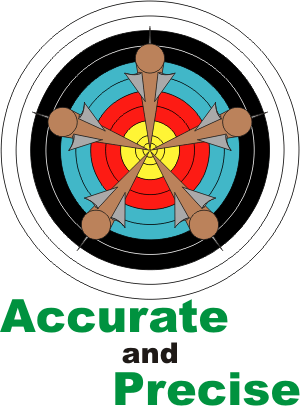### Accuracy

An accurate measurement is one which is close to the true value. Accuracy is a measure of how much error there is in the measurement and therefore the results..

To improve accuracy take an average of repeats of the experiment. This reduces the effect of random error but has no effect on systematic error.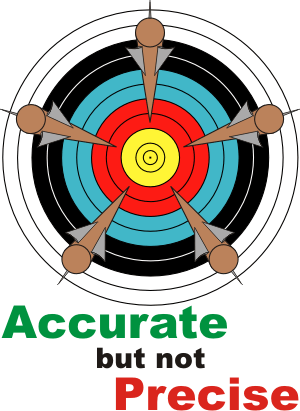### Precision

The precision of a measurement is determined by the limits of the scale on the instrument being used. Precision is related to the smallest scale division on the measuring instrument that you are using. It may be the case that a set of precise measurements has very little spread about the mean value.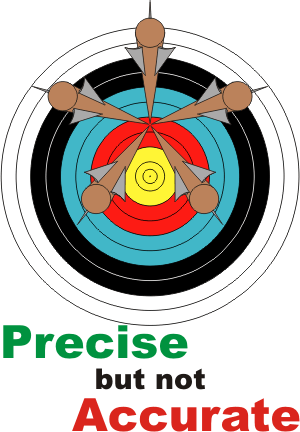• For example, using a ruler with a millimetre scale on it to measure the thickness of a book will give greater precision than using a ruler that is only marked in centimetres.

### Fair test

A fair test is one in which only the independent variable has been allowed to affect the dependent variable.

• For example: A fair test can usually be achieved by keeping all other variables constant.

### Data

This refers to a collection of measurements.

• For example: Data can be collected for the volume of a gas or the type of rubber.

### Datum

The singular of data.

### Value

A value is a numerical quantity measured

### Table

Tables used to organise and record observational data. The headings in a table are a description of the numbers to be written underneath. It is usual for the Independent variable to be the left hand column and the Dependant variable to be the right hand column.

Depending on the investigation there may be additional columns in the middle of the table if the dependant variable is calculated from other measurements. It is important to record all observations made.

Example 1:

Independant Variable
(Units)
Dependant Variable
(Units)
Mean Dependant Variable
(Units)

Example 2:

Independant Variable
(Units)
Dependant Variable
(Units)
Mean Dependant Variable
(Units)

 When drawing a table for an ISA there are two marks available: Independant variable Headings Units Dependant variable Headings Units The headings should be a full description of the measurements eg. Don't just write 'volume' when you are measuring the volume of water collected in 24hrs. In the ISA you are not required to provide space for repeats to gain full marks, nor are you required to put any numbers into the table as you won't have conducted your preliminary test at this point. Don't forget to HU HU!

## Averages

There are 3 types of Averages:

### Mean

NOTE: Most commonly used average in Science

The mean reduces the effects of random error. When calculating the mean we should disregard (ignore) any anomalous results

To calculate the mean of a data set, first sum them (add them up) then divide by the number of numbers

For example:

Age
(yrs)
Height
(cm)
Mean Height
(cm)
11 137 123 132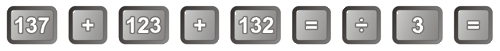Don't forget to press equals after adding up the numbers or putting the sum into brackets.

### Median

NOTE: Rarely used in Science

The Median is the middle number. Simply sort the numbers into size order the locate the middle number.

### Modal

NOTE: Rarely used in Science

The Modal is the most common number. The word 'mode' comes from the french for 'fashion'.

## Graphs and Charts

Depending on the type of variables in our data we use different methods of graph to make seeing a relationship easier.

The Independant variable goes on the x-axis and the Dependant variable goes on the y-axis (obviously this does not apply to a pie chart which doesn't have axis!)

When deciding on a scale, think of the values of coins. Make your scale go up in either 1, 2, 5, 10, 20, 50 or 100's.

To decide which value to go up in:

1. Find the largest number to be plotted on the axes
2. Count up the maximum number of squares that can be used
3. Divide the largest number by the maximum number of squares
4. Then round up to the nearest coin value

It is also sensible to use the 'coin values' when dealing with numbers less than 1. For example going up in 0.20's could be a good scale.

Going up in 4's can also be a good scale as it is easy to plot the numbers in between divisions.

If your graph is less than half a page in size, then go up in 2 squares thereby doubling your scale. Start in bottom left corner of the page to give yourself the most space possible, when it comes to graphs - big is better.

### Bar Chart

NOTE: Rarely used in Science

Used to display data where one variable is Categoric (or Ordered) and the other Continuous. This can normally be easily recognised as one set of word values and one set of numerical values.

There are gaps between the bars as the edges of the bars bare no relationship to the next bar. The bars must be of equal width.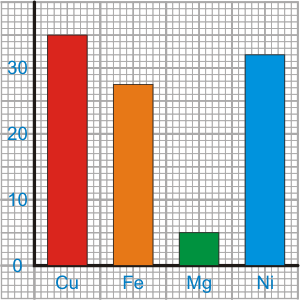• For example: Time taken for a metal bar to conduct heat along its length

 When drawing a bar chart for an ISA there are four marks available: X axis The bars are of equal width and not touching with the graph being more than ½ a page Axis is labelled -This comes from the results table heading The bars are correctly labelled Y axis Scale is linear (equally spaced values) and means the graph is more than ½ a page Axis is labelled -This comes from the results table heading Units -This comes from the results table heading Bars correctly plotted to within a millimetre

### Histogram

NOTE: Rarely used in Science

Used to display frequency (counts / tally) data between interval categories. Here there are no gaps between bars, because the left hand side of one bar is just short of the value of the right hand side of the next bar.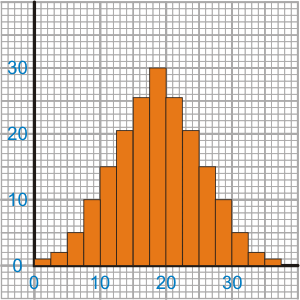• For example: Number of leaves within a specific range of length.

### Line Graph

Used to display continuous variables, ie. numeric values on each axis.

NOTE: Rarely used in Science

A scatter graph with a dot-to-dot line. This is used when there is extreme confidence in the data and there isn't likely to be an overriding trend / relationship. Theses are nearly always time related.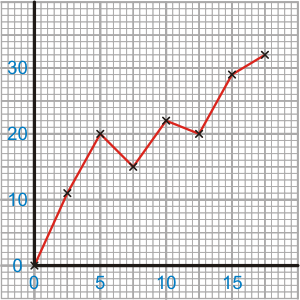• For example: Speed-Time graphs, Growth with respect to time, (Business Profits)

### Pie Chart

NOTE: Rarely used in Science

Used to display the relative proportions of a total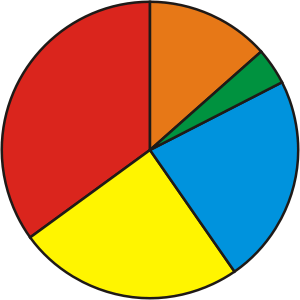• For example: The relative proportions of sources of background radiation

### Scatter Graph

NOTE: Most commonly used graph in Science

Used to display data with two sets of continuous variables. This can be easily recognised as two sets of numerical values.

Points are plotted and a smooth 'Line of Best Fit' is drawn. This shows the general trend and averages out any errors in the data. The 'Line of best Fit' on a scatter graph is the representation of our best guess at the true values.

The line could be straight ie. linear, or equally correct is a curved line. It is a judgement to be made when looking at the data points. Because of this, there should be at least 5 data points to enable the trend to be correctly identified.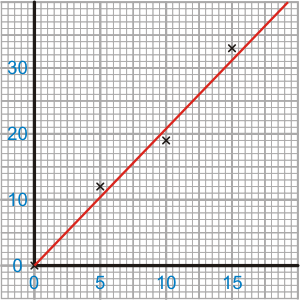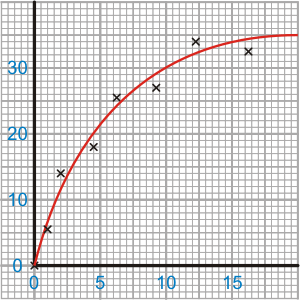• For example: Weight against Mass, Temperature against Time or Count Rate against Time

The independent variable is plotted on the x-axis (the axis at the bottom of the graph). The dependant variable is plotted on the y-axis (the axis at the size of the graph)

Common shapes of lines are:

 When drawing a scatter graph for an ISA there are four marks available: X axis Scale is linear (equally spaced values) and means the graph is more than ½ a page Axis is labelled-This comes from the results table heading Units-This comes from the results table heading Y axis Scale is linear (equally spaced values) and means the graph is more than ½ a page Axis is labelled-This comes from the results table heading Units-This comes from the results table heading Points correctly plotted to within a millimetre - You must use a sharp pencil for this There is a thin (use a sharp pencil), smooth 'Line of Best Fit'-draw the 'Line of Best Fit' with a ruler if the points are close to being a straight line NB: If there is no pattern to the data, such that a 'Line of Best Fit' can not be drawn, then write on the graph: 'There is no correlation in the results' to gain the mark.

### Linear Scale

A linear scale is one where each centimetre on the axes equals the same amount. For example, if the scale goes up in 10s, for every one centimetre square, then the scale is linear.

## Errors

### Random Error

Random error, also known as experimental error, affect each measurement differently. They are normally due to a variation in a control variables that we are unable to keep exactly the same. For example the room temperature during the experiment - it is approximately the same, but small variation always occur.

Random errors are represented on a scatter graph by the distance from the 'Line of Best Fit'. In the diagram below the green lines show the error. It is assumed that the error in measuring the independent variable is low compared to that of the dependant variable - hence the green lines are vertical. If the points are all close to the 'Line of Best Fit' then we can say that our results are good as the error is minimal.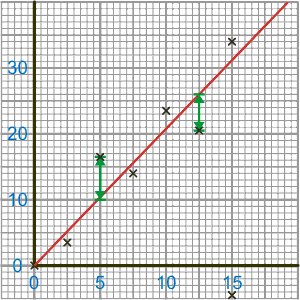Random errors may be detected and compensated for by taking a large number of readings.

• For example: Random errors may be caused by human error, a faulty technique in taking the measurements, or by faulty equipment.

### Systematic Error

These cause readings to be spread about some value other than the true value; in other words, all the readings are shifted one way or the other way from the true value. Systematic errors occur to the same amount every time that you take a particular reading and therefore, if detected, can be corrected.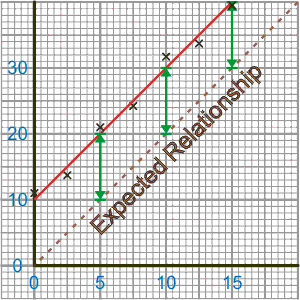• For example: A systematic error occurs when using a wrongly calibrated instrument.

### Zero Error

These are a type of systematic error. They are caused by measuring instruments that have a false zero.

• For example: A zero error occurs when the needle on an ammeter fails to return to zero when no current flows, or when a top-pan balance shows a reading when there is nothing placed on the pan.

### Anomaly

An anomaly is a point significantly out of place compared to the other results. This is normally due to a misreading or a mistake in the recording / plotting of results. These should be ignored both when calculating a mean drawing a 'Line of Best Fit' as they are clearly wrong - beyond the realms of random error.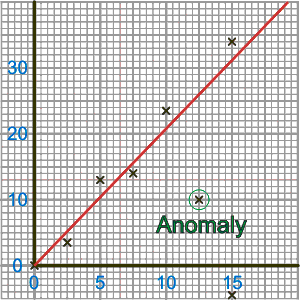It is common practice to circle any anomalies, both on the graph and in a table of results.

### Parallax

Parallax error is caused when reading from a needle (analogue) meter, when not looking straight on at the meter.

High end analogue meters have a mirrored back to eliminate parallax error. When reading such a meter, make sure that the reflection of the needle is behind the needle itself - this means that you must be looking straight on to the meter.

Digital meters do not suffer from this effect as it does not matter which angle you look at the display, it will always show the same reading.

### Conclusion

The Conclusion is the final part of the analysis. It states what the results show and should answer the aim. It should be backed up with evidence from your results and then explained using Scientific Theory.

Remember to use:

 Point - Evidence - Explain

 In an ISA there are normally 3 marks available for the conclusion. You need to be as detailed as you can. A good start is to describe the direction of the 'Line of Best Fit' and then its shape. Half of the marks are for backing up your claims with numerical data from your results as a quantitative statement.

How to write a relationship

### Evaluation

The Evaluation describes how sure you are of your conclusion and attempts to explain where errors came from. Also included is the ways in which you could improve the experiment.

 In an ISA there could be questions asking how you made your results fair, accurate and reliable.

### Preliminary Test

The first part of an experiment in which the equipment is used to decide things about the actual experiment. For example:

### Range

The range of a variable is the difference between the largest and the smallest values for a variable.

It is better to have as large a range as is possible for the independent variable, as this normally gives the biggest change in the dependant variable. This makes identifying any patterns that much easier and reduces the effect of any errors.

When quoting a range give the actual values used.

### Interval

The interval is the gap between the chosen values for the independent variable. This should be chosen in conjunction with the range to produce at least 5 different readings.

While in most investigations the interval is constant, it is only a rough guide to make sure that we have data points spaced throughout the range and therefore the graph.

We sometimes may vary the interval to concentrate on a particular point in the relationship. This is especially true when a we have a 'U-shaped' Line of Best Fit. We would normally be interested finding the point at which the line turned. Although this may form a secondary experiment.

### Reliability

Reliability is a measure of how much you can trust your conclusion drawn from your results.

The results of an investigation may be considered reliable if the results can be repeated. If someone else can carry out your investigation and get the same results, then your results are more likely to be reliable.

You may not need to do repeats if:

• A second measurement matches exactly the first one
• The first set of measurements lie very close to a line of best fit. To know this you would need to plot the first set measurements before packing away.

### Repeatable

Results are said to be repeatable if you can get the same results when you redo your experiment.

### Reproducible

Results are said to be reproducible if someone else can get the same results when they redo your experiment.

### Validity

Data is only valid for use in coming to a conclusion if the measurements taken are affected by a single independent variable only. Data is not valid if for example a fair test is not carried out or there is observer bias.

• For example: In an investigation to find the effect on the rate of a reaction when the concentration of the acid is changed, it is important that concentration is the only independent variable. If, during the investigation, the temperature also increased as you increased the concentration, this would also have an effect on your results and the data would no longer be valid.

### Sensitivity

The sensitivity of an instrument refers to the smallest change in a value that can be detected.

• For example, bathroom scales are not sensitive enough to detect the weekly changes in the mass of a baby, whereas scales used by a midwife are sensitive enough to permit a growth chart to be plotted.

### Resolution

The resolution of a measuring instrument is the smallest measurement it can measure, ie the smallest division marked on the scale, or possibly half of a division.

You can tell if you have used an measuring division with appropriate resolution if the difference between readings is much greater (5 times bigger) than the resolution used.

### Calibration

This involves fixing known points and then marking a scale on a measuring instrument, using these fixed points. It can also be done to an electronic sensor's output.

The sensor would need to be placed in a known condition, which was measured by another device. You would then record the known condition and the sensors output. Finally you would mark the known condition on a scale that the sensor's output will be measured on.

• For example: To mark the lines on a thermometer, put the thermometer in just melting ice - which we know is at 0°C. Then mark 0°C on the thermometer at the height of the fluid. Next put the thermometer in just boiling water, mark this as 100°C. Then draw 100 equally spaced marks in between, to represent each degree

### Numerical

A numerical value is one that is a number.

### Quantitative

Quantitative statements are those for which a numerical value representing is expressed. It is quantitative statements that scientist try to produce. It is how you should justify your conclusions, as the evidence part of:

 Point - Evidence - Explain

### Optimum

The Optimum value is the best value to use. It is used to describe the top of a peak or the bottom of a trough on a graph - depending on what is best: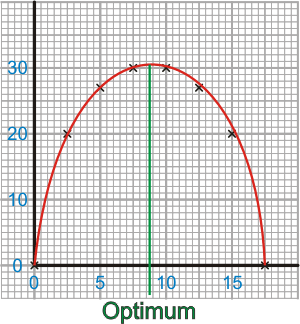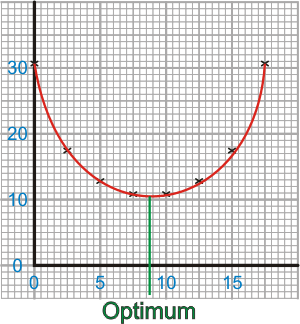Any value less than the optimum produces not as good a result and any value greater than the optimum produces not as good a result.